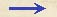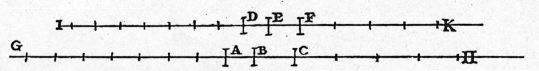Discorsi Propositions 1/01-th-01 Discorsi Proposition1/01-th-01THEOREMA, PROPOSITIO I. THEOREM 1, PROPOSITION 1Si mobile aequabiliter latum eademque cum velocitate duo pertranseat spatia, tempora lationum erunt inter se ut spatia peracta. If a moving particle, carried uniformly at a constant speed, traverses two distances the time-intervals required are to each other in the ratio of these distances. Pertranseat enim mobile aequabiliter latum eadem cum velocitate duo spatia AB, BC, et sit tempus motus per AB, DE; tempus vero motus per BC esto EF: dico, ut spatium AB ad spatium BC, ita esse tempus DE ad tempus EF. Protrahantur utrinque spatia et tempora versus G, H et I, K, {10} et in AG sumantur quotcunque spatia ipsi AB aequalia, et totidem tempora in DI, tempori DE similiter aequalia; et rursus in CH sumantur secundum quamcunque multitudinem spatia ipsi CB aequalia, et totidem tempora in FK, tempori EF aequalia: erunt iam spatium BG et tempus EI aeque multiplicia spatii BA et temporis ED iuxta quamcunque multiplicationem accepta, et similiter spatium HB et tempus KE spatii CB temporisque EE aeque multiplicia in qualibet multiplicatione. Et quia DE est tempus lationis per AB, erit totum EI tempus totius BG, cum motus ponatur aequabilis sintque in EI tot tempora ipsi DE aequalia quot sunt in BG spatia aequalia BA; et similiter concludetur, KE esse tempus lationis per HB.Cum autem {20} motus ponatur aequabilis, si spatium GB esset aequale ipsi BH, tempus quoque IE tempori EK foret aequale; et si GB maius sit quam BH, etiam IE {193} quam EK maius erit; et si minus, minus.Sunt itaque quatuor magnitudines, AB prima, BC secunda, DE tertia, EF quarta, et primae et tertiae, nempe spatii AB et temporis DE, sumpta sunt aeque multiplicia iuxta quamcunque multiplicationem tempus IE et spatium GB; ac demonstratum est, haec vel una aequari, vel una deficere, vel una excedere, tempus EK et spatium BH, aeque multiplicia scilicet secundae et quartae: ergo prima ad secundam, nempe spatium AB ad spatium BC, eandem habet rationem quam tertia et quarta, nempe tempus DE ad tempus EF: quod erat demostrandum. Let a particle move uniformly with constant speed through two distances AB, BC, and let the time required to traverse AB be represented by DE; the time required to traverse BC, by EF; then I say that the distance AB is to the distance BC as the time DE is to the time EF. Let the distances and times be extended on both sides towards G, H and I, K; let AG be divided into any number whatever of spaces each equal to AB, and in like manner lay off in DI exactly the same number of time-intervals each equal to DE. Again lay off in CH any number whatever of distances each equal to BC; and in FK exactly the same number of time-intervals each equal to EF; then will the distance BG and the time EI be equal and arbitrary multiples of the distance BA and the time ED; and likewise the distance HB and the time KE are equal and arbitrary multiples of the distance CB and the time FE. And since DE is the time required to traverse AB, the whole time EI will be required for the whole distance BG, and when the motion is uniform there will be in EI as many time-intervals each equal to DE as there are distances in BG each equal to BA; and likewise it follows that KE represents the time required to traverse HB.Since, however, the motion is uniform, it follows that if the distance GB is equal to the distanc BH, then must also the time IE be equal to the time EK; and if GB is greater than BH, then also IE will be greater than EK; and if less, less.There {193} are then four quantities, the first AB, the second BC, the third DE, and the fourth EF; the time IE and the distance GB are arbitrary multiples of the first and the third, namely of the distance AB and the time DE. But it has been proved that both of these latter quantities are either equal to, greater than, or less than the time EK and the space BH, which are arbitrary multiples of the second and the fourth. Therefore the first is to the second, namely the distance AB is to the distance BC, as the third is to the fourth, namely the time DE is to the time EF. Q. E. D.Discorsi Propositions 1/01-th-01 Discorsi Proposition1/01-th-01